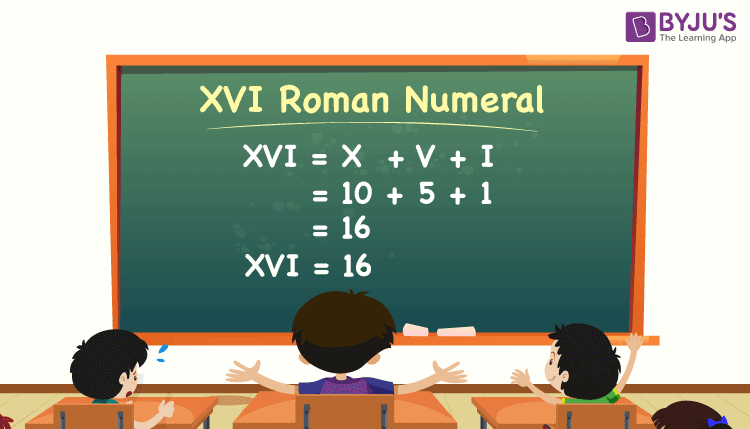# XVI Roman Numerals

XVI Roman numeral value in numbers is 16. Before learning how to convert roman numerals to numbers, first, understand what roman numerals are. Roman numerals are a special type of numerical notations that were first used by the Romans. This is an additive and subtractive system, where letters indicate specific base numbers in the number system, and are denoted using a different combination of letters or symbols. This system represents numbers using varied or particular letters from the Latin alphabet. The modern method employs seven characters; each of these characters bears a fixed integer value, as given below:

 Character (or Letter) I V X L C D M Numerical Value 1 5 10 50 100 500 1000

Let’s understand the meaning of the XVI Roman numeral and the conversion of the XVI Roman numeral to a number in different ways.

## XVI Value in Roman Numerals

The numerical value of the roman numeral XVI is 16. Thus, XVI means sixteen in natural numbers. As X means 10, V means 5 and I means 1, so by adding all these together, we get the numerical value of XVI as 16.### XVI Roman Numerals Conversion

In roman numerals, we can find the value of XVI using the addition method. Let us write the numerical value of each character or letter of roman numerals here. Then, add the required numerals to get the value of XVI. This addition can be done in many ways, as illustrated below:

Method 1: In this method, write the numerical value of each letter and add them to get the result.

XVI = 10 + 5 + 1 = 16

Method 2: In this method, the numerical values of groups of letters are considered for addition, such as:

XVI = (X) + (VI) = 10 + 6 = 16

 XVI Roman numerals in numbers = XVI = 16

The above methods are used to find the numerical value of all the roman numerals to a possible extent.

Also, check: XXXVII Roman numerals

### How to convert Roman numerals to Numbers?

Before going to the conversion of roman numbers to numbers, first, understand the representation of this system of numbers in detail. Consider the following sequence of roman numerals:

I, II, III, IV, V, VI, VII, VIII, IX, X, XI, XII, XIII, XIV, XV, XVI, XVII, XVIII, XIX, XX

From this, we can write the following basic rules for the conversion of roman numerals into numerical values.

• When a bigger letter is succeeded by a smaller letter, we should subtract the corresponding numerical values. For example, the value of IV can be derived as V – I = 5 – 1 = 4, where V is the bigger number.
• Thus, the notation IV can be read as “one less than five, i.e. 4”.
• Consider IX, where X is the bigger number. So, IX = X – I = 10 – 1 = 9. Also, the notation IX can be read as “one less than ten, i.e. 9”.
• When a bigger letter is preceded by a smaller letter, we should add the corresponding numerical values. For example, VI is the roman numeral, where the bigger number V is succeeded by the smaller number I. So, add the numerals, VI = V + I = 5 + 1 = 6.
• The notations VI and XI can be read as “one more than 5, i.e. 6” and “one more than 10, i.e. 11”, respectively.

Get roman numerals conversion along with solved examples here.

The table below shows the convention values that will help us to write numbers in roman numerals, and vice versa accurately.

 Units Tens Hundreds Thousands 1 I X C M 2 II XX CC MM 3 III XXX CCC MMM 4 IV XL CD 5 V L D 6 VI LX DC 7 VII LXX DCC 8 VIII LXXX DCCC 9 IX XC CM

## Video Lesson on Roman Numerals### Numbers nearer to XVI

The numbers which are close to, less than and greater than XVI are given below, along with conversions.

XIII roman numeral = 10 + 1 + 1 + 1 = 13

XIV roman numeral = 10 + 4 = 14

XV roman numeral = 10 + 5 = 15

XVI roman numeral = 10 + 5 + 1 = 16

XVII roman numeral = 10 + 5 + 1 + 1 = 17

XVIII roman numeral = 10 + 5 + 1 + 1 + 1 = 18

XIX roman numeral = 10 + 9 = 19

XX roman numeral = 10 + 10 = 20

XXI roman numeral = 10 + 10 + 1 = 21

XXII roman numeral = 10 + 10 + 1 + 1 = 22

XXIII roman numeral = 10 + 10 + 1 + 1 + 1 = 23

XXIV roman numeral = 10 + 10 + 4 = 24

XXV roman numeral = 10 + 10 + 5 = 25

XXVI roman numeral = 10 + 10 + 5 + 1 = 26